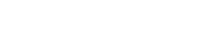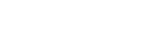# Working with Lombok in Java

Ana Peterlić, Senior Software Developer
14.03.2023.In this article, we’ll do a quick introduction to Project Lombok - the library that can help us reduce repetitive and boilerplate code in our Java classes.

Sometimes, even a simple POJO (Plain Old Java Object) can become really complex and unreadable. Suppose we have a class Order that has ten fields.

Now, if we want to embrace encapsulation, we should provide getters and setters (for non-final fields) for our private instance variables.

Additionally, we should consider overriding equals() and hashCode() methods. To override these methods, we should follow the contract provided by Oracle.

Finally, to have a nice output in the console, we should override the toString() method.

Our class, with only basic components, can end up looking like this:

`public class Order`

`{`

`   private Long id;`

`   private String name;`

`   private UUID code;`

`   private BigDecimal amount;`

`   private BigDecimal vat;`

`   private OrderType type;`

`   private boolean paid;`

`   private PaymentType paymentType;`

`   private LocalDate created;`

`   private LocalDate updated;`

`   public Long getId()`

`   {`

`       return id;`

`   }`

`   public void setId(Long id)`

`   {`

`       this.id = id;`

`   }`

`   public String getName()`

`   {`

`       return name;`

`   }`

`   public void setName(String name)`

{

`       this.name = name;`

`   }`

`   public UUID getCode()`

`   {`

`       return code;`

`   }`

`   public void setCode(UUID code)`

`   {`

`       this.code = code;`

`   }`

`   public BigDecimal getAmount()`

`   {`

`       return amount;`

`   }`

`   public void setAmount(BigDecimal amount)`

`   {`

`       this.amount = amount;`

`   }`

`   public BigDecimal getVat()`

`   {`

`       return vat;`

`   }`

`   public void setVat(BigDecimal vat)`

`   {`

`       this.vat = vat;`

`   }`

`   public OrderType getType()`

`   {`

`       return type;`

`   }`

`   public void setType(OrderType type)`

`   {`

`       this.type = type;`

`   }`

`   public boolean isPaid()`

`   {`

`       return paid;`

`   }`

`   public void setPaid(boolean paid)`

`   {`

`       this.paid = paid;`

`}`

`   public PaymentType getPaymentType()`

`   {`

`       return paymentType;`

`   }`

`   public void setPaymentType(PaymentType paymentType)`

`   {`

`       this.paymentType = paymentType;`

`   }`

`   public LocalDate getCreated()`

`   {`

`       return created;`

`   }`

`   public void setCreated(LocalDate created)`

`   {`

`       this.created = created;`

`   }`

`   public LocalDate getUpdated()`

`   {`

`       return updated;`

`   }`

`   public void setUpdated(LocalDate updated)`

`   {`

`       this.updated = updated;`

`   }`

`   @Override`

`   public boolean equals(Object o)`

`   {`

`       if (this == o) return true;`

`       if (o == null || getClass() != o.getClass()) return false;`

`       Order that = (Order) o;`

`       return paid == that.paid && Objects.equals(id, that.id) && Objects.equals(name, that.name) && Objects.equals(code, that.code) && Objects.equals(amount, that.amount) && Objects.equals(vat, that.vat) && type == that.type && paymentType == that.paymentType && Objects.equals(created, that.created) && Objects.equals(updated, that.updated);`

`   }`

`   @Override`

`   public int hashCode()`

`   {`

`       return Objects.hash(id, name, code, amount, vat, type, paid, paymentType, created, updated);`

`   }`

`   @Override`

`   public String toString()`

`   {`

`       return "Order{" +`

`               "id=" + id +`

`               ", name='" + name + '\'' +`

`               ", code=" + code +`

`               ", amount=" + amount +`

`               ", vat=" + vat +`

`               ", type=" + type +`

`               ", paid=" + paid +`

`               ", paymentType=" + paymentType +`

`               ", created=" + created +`

`               ", updated=" + updated +`

`               '}';`

`   }`

`}`

Using only the basic methods, our class ended up having nearly 200 lines of code. In addition, we haven't even added constructors. They would take a few lines as well.

### Include Lombok

To use Lombok in our project, we need to add the following dependency:

`<dependency>`

`     <groupId>org.projectlombok</groupId>`

`     <artifactId>lombok</artifactId>`

`     <optional>true</optional>`

`</dependency>`

Additionally, depending on the IDE, we need to enable annotation processing inside the Annotation Processor settings:

### Lombok Annotations

One of the things that make the Lombok library so popular is the fact that it provides annotations that help us reduce boilerplate code.

Now, let’s go through the most used Lombok annotations.

#### @Data

The @Data annotation is probably one of the most used Lombok annotations.

It includes several other annotations:

• @Getter (generates getter methods)
• @Setter (generates setter methods)
• @ToString (adds the toString() method in our class)
• @EqualsAndHashCode (overrides the equals() and the hashCode() methods in our class)

Each of the annotations mentioned above can be used separately. For instance, if we want Lombok to generate only getters for our instance variables, we could use @Getter annotation instead.

Using the @Data annotation, we can omit getters and setters, and basic methods from our class. Lombok will generate them for us.

Now, let’s go back to our Order class. Using Lombok, we can reduce our code to just fields and one annotation:

@Data

`public class Order`

`{`

`   private Long id;`

`   private String name;`

`   private UUID code;`

`   private BigDecimal amount;`

`   private BigDecimal vat;`

`   private OrderType type;`

`   private boolean paid;`

`   private PaymentType paymentType;`

`   private LocalDate created;`

`}`

We increased the readability of the code inside our class.

Furthermore, we can access fields using generated getters and setters:

`Order order = new Order();`

`order.setName("Apple");`

`order.setCode(UUID.randomUUID());`

`System.out.printf("Name: %s %n", order.getName());`

`System.out.println(order);`

#### @NoArgsConstructor, @RequiredArgsConstructor, and @AllArgsConstructor

Lombok also provides annotations for constructor generation.

We could use @NoArgsConstructor if we need a constructor without arguments.

Likewise, the @AllArgsConstructor annotation will generate a constructor that receives all instance variables as arguments.

The @RequiredArgsConstructor will include final fields that are not initialized as well as the fields annotated with @NonNull Lombok annotation.

We could use the @NonNull annotation on the fields, or constructor or method parameters. Lombok will generate a null check for the fields annotated with such an annotation. If the field is null, Lombok will throw the NullPointerException.

#### @Builder

The Builder Pattern is one of the most used creational patterns. We usually use it to create new objects. If our class has many instance variables of the same type, we could accidentally pass the wrong value in our constructor. Builder pattern helps us prevent this problem.

Using the @Builder annotation, we can easily implement the Builder Pattern in our class:

`@Builder`

`@Data`

`public class Order{`

`   // instance variables`

`}`

Next, we could use the builder to create our object:

`Order order = Order.builder().name("Apple").code(UUID.randomUUID()).build();`

The @Builder annotation replaces the following code in our class:

`public static final class builder`

`{`

`   private String name;`

`   private UUID code;`

`   private Set<Product> products;`

`   private BigDecimal amount;`

`   private BigDecimal vat;`

`   private OrderType type;`

`   private boolean paid;`

`   private PaymentType paymentType;`

`   private User user;`

`   private Payment payment;`

`   private LocalDate created;`

`   private LocalDate updated;`

`   public static builder builder()`

`   {`

`       return new builder();`

`   }`

`   public builder withName(String name)`

`   {`

`       this.name = name;`

`       return this;`

`   }`

`   public builder withCode(UUID code)`

`   {`

`       this.code = code;`

`       return this;`

`   }`

`   public builder withProducts(Set<Product> products)`

`   {`

`       this.products = products;`

`       return this;`

`   }`

`   public builder withAmount(BigDecimal amount)`

`   {`

`       this.amount = amount;`

`       return this;`

`   }`

`   public builder withVat(BigDecimal vat)`

`   {`

`       this.vat = vat;`

`       return this;`

`   }`

`   public builder withType(OrderType type)`

`   {`

`       this.type = type;`

`       return this;`

`   }`

`   public builder withPaid(boolean paid)`

`   {`

`       this.paid = paid;`

`       return this;`

`   }`

`   public builder withPaymentType(PaymentType paymentType)`

`   {`

`       this.paymentType = paymentType;`

`       return this;`

`   }`

`   public builder withUser(User user)`

`   {`

`       this.user = user;`

`       return this;`

}

`   public builder withPayment(Payment payment)`

`   {`

`       this.payment = payment;`

`       return this;`

`   }`

`   public builder withCreated(LocalDate created)`

`   {`

`       this.created = created;`

`       return this;`

`   }`

`   public builder withUpdated(LocalDate updated)`

`   {`

`       this.updated = updated;`

`       return this;`

`   }`

`   public Order build()`

`   {`

`       Order order = new Order();`

`       order.setName(name);`

`       order.setCode(code);`

`       order.setProducts(products);`

`       order.setAmount(amount);`

`       order.setVat(vat);`

`       order.setType(type);`

`       order.setPaid(paid);`

`       order.setPaymentType(paymentType);`

`       order.setUser(user);`

`       order.setPayment(payment);`

`       order.setCreated(created);`

`       order.setUpdated(updated);`

`       return order;`

`   }`

`}`

#### @Log

In case we want to use a library for logging our code, we could simply use the @Log annotation. Lombok will generate a variable named log which we could use for logging.

Furthermore, we could include a special library for logging. For example, if we want to use the slf4j library, we could use @Slf4j annotation:

`@Slf4j`

`@Service`

`public class DefaultOrderService implements OrderService`

`{`

`   private final OrderRepository orderRepository;`

`   public DefaultOrderService(OrderRepository orderRepository)`

`   {`

`       this.orderRepository = orderRepository;`

`   }`

`   @Override`

`   public void add(Order order)`

`   {`

`       log.info("Adding new order: {}", order);`

`       orderRepository.save(order);`

`   }`

`}`

The annotation replaces our Logger instance variable:

`private static final org.slf4j.Logger log = org.slf4j.LoggerFactory.getLogger(DefaultOrderService.class);`

### Pros and Cons

To sum up, let’s list the pros and cons of Project Lombok library.

#### Pros

The main advantage of using Lombok is reducing the number of lines in our code. We can reduce getters, setters and other basic methods our class should have. Therefore, using Lombok increases code readability.

#### Cons

On the other hand, using Lombok has its downsides. First and foremost, our code depends on the external library. The library may not follow the changes made in Java, or it may not support some new features Java offers.

When we use Lombok with ORM (for instance Hibernate), the number of annotations on the class level may increase. Furthermore, using Lombok with Hibernate may cause StackOverflowError in situations when we have a bidirectional association and we want to print an object in the console. Hence, to fix this problem, we would need to override the toString() method by ourselves.

Lastly, Lombok doesn’t have the ability to detect the constructors (not generated by Lombok) of parent classes.

### Conclusion

In this article, we looked into the Project Lombok library and explained the basic annotations.